SSC  >  Test: Fluid Mechanics- 2

# Test: Fluid Mechanics- 2

Test Description

## 20 Questions MCQ Test Mock Test Series of SSC JE Civil Engineering | Test: Fluid Mechanics- 2

Test: Fluid Mechanics- 2 for SSC 2023 is part of Mock Test Series of SSC JE Civil Engineering preparation. The Test: Fluid Mechanics- 2 questions and answers have been prepared according to the SSC exam syllabus.The Test: Fluid Mechanics- 2 MCQs are made for SSC 2023 Exam. Find important definitions, questions, notes, meanings, examples, exercises, MCQs and online tests for Test: Fluid Mechanics- 2 below.
Solutions of Test: Fluid Mechanics- 2 questions in English are available as part of our Mock Test Series of SSC JE Civil Engineering for SSC & Test: Fluid Mechanics- 2 solutions in Hindi for Mock Test Series of SSC JE Civil Engineering course. Download more important topics, notes, lectures and mock test series for SSC Exam by signing up for free. Attempt Test: Fluid Mechanics- 2 | 20 questions in 12 minutes | Mock test for SSC preparation | Free important questions MCQ to study Mock Test Series of SSC JE Civil Engineering for SSC Exam | Download free PDF with solutions
 1 Crore+ students have signed up on EduRev. Have you?
Test: Fluid Mechanics- 2 - Question 1

### The correct statement about ideal fluid is:

Detailed Solution for Test: Fluid Mechanics- 2 - Question 1

A fluid is said to be ideal if it assumed to be both incompressible and non-viscous, its bulk modulus is infinite. An ideal fluid is a fluid that has several properties including the fact that it is:

Incompressible - the density is constant

Non-viscous - (Inviscid) fluid has no internal friction.

Test: Fluid Mechanics- 2 - Question 2

### Specific gravity of water is 1.0 which is reported at a temperature of:

Detailed Solution for Test: Fluid Mechanics- 2 - Question 2

Specific gravity of water is 1.0 which is reported at a temperature of 4°C. Specific gravity is a measure of density of liquid.

Some interesting facts about Specific gravity:

Specific gravity of water is actually a density of water in gram per cubic centimetre.

Test: Fluid Mechanics- 2 - Question 3

### Quick sand comes under:

Detailed Solution for Test: Fluid Mechanics- 2 - Question 3

Newtonian Fluids: Air, water, mercury, glycerine, kerosene and other engineering fluids under normal circumstances.

Pseudoplastic: Fine particle suspension, gelatine, blood, milk, paper pulp, polymeric solutions such as rubbers, paints.

Dilatant fluids: Ultra fine irregular particle suspension, sugar in water, aqueous suspension of rice starch, quicksand, butter printing ink.

Ideal plastics or Bingham fluids: Sewage sludge, drilling muds.

Viscoelastic fluids: Liquids solid combination in pipe flow, bitumen, tar, asphalt, polymerized fluids with drag reduction features.

Thixotropic: Printer’s ink, crude oil, lipstick, certain paints and enamels.

Rheopectic fluids: Very rare liquid - solid suspensions, gypsum suspension in water and bentonite solutions.

Test: Fluid Mechanics- 2 - Question 4

Discharge Q in a triangular weir varies as:-

Detailed Solution for Test: Fluid Mechanics- 2 - Question 4

Discharge through a triangular notch/weir is given by: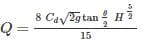Where,

H = height of liquid above apex of the notch

θ = Angle of notch

Cd = Coefficient of discharge

Test: Fluid Mechanics- 2 - Question 5

For a uniform flow with depth of 0.6 m and Froude number of 2.0 in a rectangular channel, the specific energy will be -

Detailed Solution for Test: Fluid Mechanics- 2 - Question 5

Froude number=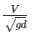Where,

V = velocity of flow through the rectangular channel

D = depth of flow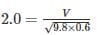V=4.85m/s

Specific Energy (E) is given by =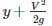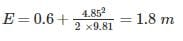Test: Fluid Mechanics- 2 - Question 6

The major loss of hydraulic energy in pipe flow occurs in long pipe due to:-

Detailed Solution for Test: Fluid Mechanics- 2 - Question 6

There are generally two types of losses occur in a pipe flow problem:

a) Major loss: Major head loss occurs due to friction, which is given by: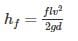Where f = friction factor=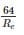1. Sudden enlargement

2. Sudden contraction

3. Due to pipe bends

4. head loss at the entrance and exit of pipe etc.

Test: Fluid Mechanics- 2 - Question 7

The eddy viscosity for turbulent flow is

Detailed Solution for Test: Fluid Mechanics- 2 - Question 7

1. Flows become turbulent due to differences in velocity inside them, and the flow resistance increases. This resistive force acting on flows due to turbulence is called the Reynolds stress. This is an imaginary force that appears when flow fields are seen with coarse graining by time-averaging.

2. Viscosity, the proportionality constant of viscous force, is a physical quantity dependent on the type of material, while eddy viscosity is a physical quantity dependent on the state of flow.

Test: Fluid Mechanics- 2 - Question 8

For a vacuum pressure of 4.5 m of water, the equivalent absolute pressure is:

Detailed Solution for Test: Fluid Mechanics- 2 - Question 8

Absolute pressure = gauge pressure + local atmospheric pressure

-ve gauge pressure = vacuum pressure = 4.5 m of water

Local atmospheric pressure of water = 10.3 m of water

Equivalent absolute pressure = -4.5 + 10.33 = 5.83 m of water

Test: Fluid Mechanics- 2 - Question 9

In a lock gate, the reaction between two gates is

Detailed Solution for Test: Fluid Mechanics- 2 - Question 9

The lock gates are provided in navigation chambers to change a water level in a canal or river in navigation. There are two sets of gates, one set on either side of the chamber.

In a lock gate, the reaction between the two gates (R) is given by: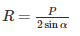P = Resultant water pressure on the lock gate

α = Inclination of the gate with the normal to the side of the lock.

Test: Fluid Mechanics- 2 - Question 10

An orifice is said to be large, if

Detailed Solution for Test: Fluid Mechanics- 2 - Question 10

An orifice is a small aperture through which the fluid passes. The thickness of an orifice in the direction of flow is very small in comparison to its other dimensions. An orifice is said to be large, if the available head of liquid is less than 5 times the height of orifice.

Test: Fluid Mechanics- 2 - Question 11

In order, to avoid separation in Venturi meter the angle of divergence is kept

Detailed Solution for Test: Fluid Mechanics- 2 - Question 11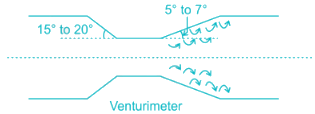In the diverging portion there is a decrease in velocity & subsequent increase in pressure. If divergence angle is very large, then back pressure will increase by great extent & eddies formation will take place resulting in flow separation. Thus, to avoid flow separation the divergence angle must not exceed more than 7° and range should be around 5 - 7°.

Test: Fluid Mechanics- 2 - Question 12

The specific speed of a pump is defined as the speed of a unit of such a size that it discharge:

Detailed Solution for Test: Fluid Mechanics- 2 - Question 12

The specific speed of a pump is defined as speed at which pump generates unit discharge under unit head.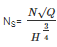where Q=discharge, H=head, Ns= specific speed, N= speed of pump.

Test: Fluid Mechanics- 2 - Question 13

The discharge over a sharp - edged rectangular notch of width w depth h is equal to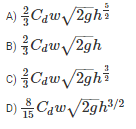Detailed Solution for Test: Fluid Mechanics- 2 - Question 13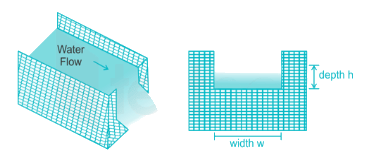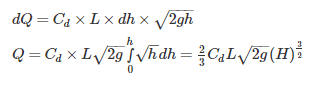Test: Fluid Mechanics- 2 - Question 14

Froude number for weak and strong jump respectively are

Detailed Solution for Test: Fluid Mechanics- 2 - Question 14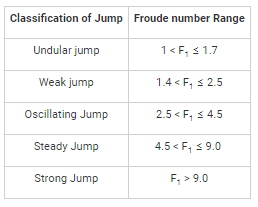Test: Fluid Mechanics- 2 - Question 15

The point at which the resultant pressure on a immersed surface acts, is known as:

Detailed Solution for Test: Fluid Mechanics- 2 - Question 15

Centre of pressure:

The point of action of total hydrostatic force on a submerged surface is called the centre of pressure. It is the point at which the resultant pressure on a immersed surface acts.

Test: Fluid Mechanics- 2 - Question 16

The sequent depth ratio in a hydraulic jump termed in a horizontal rectangular channel is 5. The Froude number of the supercritical stream is

Detailed Solution for Test: Fluid Mechanics- 2 - Question 16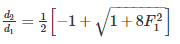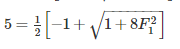F= 3.87

Test: Fluid Mechanics- 2 - Question 17

In a laminar flow in a pipe the shear stress is:

Detailed Solution for Test: Fluid Mechanics- 2 - Question 17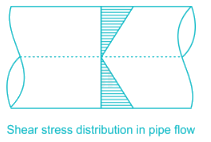Test: Fluid Mechanics- 2 - Question 18

The flow of water in a wash hand basin when it is being emptied through a central opening, is an example of

Detailed Solution for Test: Fluid Mechanics- 2 - Question 18

In a free vortex flow total mechanical energy remains constant. There is neither any energy interaction between an outside source and the flow, nor is there any dissipation of mechanical energy within the flow. The fluid rotates by virtue of some rotation previously imparted to it or because of some internal action.

Some examples are a whirlpool in a river, the rotatory flow that often arises in a shallow vessel when liquid flows out through a hole in the bottom (when water flows out from a bathtub or a wash basin), and flow in a centrifugal pump case just outside the impeller.

Test: Fluid Mechanics- 2 - Question 19

A compound pipe of diameters d1, d2 and d3 having length l1, l2 and l3 respectively is to be replaced by an equivalent pipe of uniform diameter d and length l. The size of the equivalent pipe is given by

Detailed Solution for Test: Fluid Mechanics- 2 - Question 19

For pipes in series equivalent pipe is given by: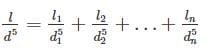For pipes in parallel equivalent pipe is given by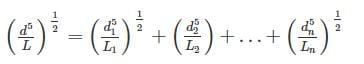Test: Fluid Mechanics- 2 - Question 20

The equation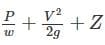Zconstant is based on the following assumptions regarding the flow of fluid

Detailed Solution for Test: Fluid Mechanics- 2 - Question 20

The equation is Bernoulli’s equation. lt is based on following assumptions:

ii) fluid is incompressible

iii) fluid is non-viscous since viscous force has been neglected: and

iv) it is applicable to points along a streamline

## Mock Test Series of SSC JE Civil Engineering

1 videos|1 docs|64 tests
 Use Code STAYHOME200 and get INR 200 additional OFF Use Coupon Code
Information about Test: Fluid Mechanics- 2 Page
In this test you can find the Exam questions for Test: Fluid Mechanics- 2 solved & explained in the simplest way possible. Besides giving Questions and answers for Test: Fluid Mechanics- 2, EduRev gives you an ample number of Online tests for practice

## Mock Test Series of SSC JE Civil Engineering

1 videos|1 docs|64 tests